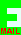## PRIZE PUZZLE FOR DECEMBER 2003

Whether or not there is a Hot Key magazine, this is the puzzle for December.

#### The Ways of Coins

In this problem, you can use coins of value 200, 100, 50, 20, 10, 5, 2 and 1 pence to make up a value of money. The number of ways you can represent a given value must be worked out. Here are some (trivial) examples. The value of 1p can only be represented in one way, namely as a single penny. The value of 2p can be represented in two ways. They are, firstly as 2 pennies and secondly as the single coin of value 2p. Three pence can also be represented in two ways: 3 single pence and 1 2p + 1 1p coin. Work out for yourself the number of ways that the value 4p can be represented. You should get the answer 3. As you might expect, the number of ways increases rapidly as the value of the money increases.

The problem this month is to find the smallest value of money that can be represented in more ways than the value of the money in pence.

You can e-mail your answer through this link (without me having to reveal my e-mail address to spam robots):The closing date is January 7th 2004.

[home] [back]